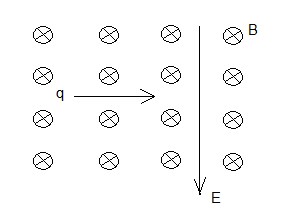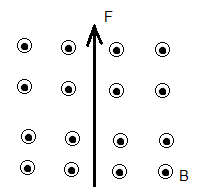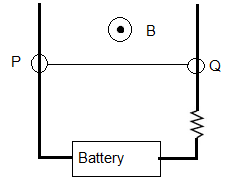# SAT Physics Practice Questions on Magnetism with Solutions

 Sat Physics subject questions on magnetic field, forces on moving charges and current carrying wires, with detailed solutions, similar to the questions in the SAT test are presented. Answers at the bottom of the page and also detailed solutions and explanations. NOTE: In what follows, the quantities in bold are vector quantities. A positively charged particle moves at a velocity v perpendicular to a magnetic field B. If the magnitude of the velocity v is doubled, then the magnitude of the force F acting on the moving charge is A) halved B) doubled C) quadrupled D) quartered E) not changed What is the direction of the force acting on a negatively charged particle moving from East to West in a magnetic field directed downward? A) directed to the right B) directed to the left C) directed upward D) directed into the page E) directed out of the page A negatively charged particle q enters from the left an area with both an electric field directed downward and a magnetic field of magnitude 6.0�10-6 T directed into the page (see figure below). What must be the magnitude of the electric field so that the particle is not deflected if the magnitude of its velocity is 2.0�105 cm/s?A) 1.2 v/m B) 3.3 � 10-11 v/m C) 1.2 � 10-2 v/m D) 3.3 � 1010 v/m E) 120 v/m Which of the following diagrams represents the directions of the forces acting on the two wires with current I flowing in the same direction as shown below?If a force F is exerted on a charged particle when the particle enters a magnetic field B at a velocity v, which of the following is always true? A) Vectors F, B and v are all perpendicular to each other B) Vectors F and B are parallel C) Vectors F and B are perpendicular D) Vectors F and v are be parallel E) Vectors B and v may perpendicular If a magnetic field B exerts a force F on a wire of length L with electric current in a magnetic field, then what value of the angle between the direction of the current i and the magnetic field B gives a maximum value for the magnitude of F? A) 180� B) 45� C) 90� D) 120� E) 0 What is the direction of the current that experiences the force F of a magnetic field B shown in the diagram below?A) Upward B) into the page C) downward D) from left to right E) from right to left In the diagram below, wire PQ is part of the closed circuit and is in a magnetic field B. If we neglect the resistance of the wires and the internal resistance of the battery, which of the following is true about the magnitude of the force acting on the wire PQ? (I) It is proportional to the magnitude of the magnetic field B (II) It is inversely proportional to R (III) It is inversely proportional to EA) (I) only B) (I), (II) and (III) C) (I) and (III) only D) (I) and (II) only E) (II) and (III) only Two parallel wires with the same current i exert forces on each other with equal magnitudes. What happens to the magnitude of this force if the current is halved? A) The magnitude is quartered B) The magnitude is halved C) The magnitude is doubled D) The magnitude is quadrupled E) The magnitude does not change In the circuit below wire PQ, with mass of 10 grams and length of 20 cm, can move up and down with negligible friction. If a uniform magnetic field B of magnitude 1 T and direction out of the page, is applied to wire PQ, what must be the direction and the smallest intensity of the current in the circuit that lifts wire PQ upward?A) Direction: from Q to P , Minimum intensity: 2 A B)Direction: from P to Q , Minimum intensity: 0.5 A C) Direction: from P to Q , Minimum intensity: 2 A D) Direction: from Q to P , Minimum intensity: 1 A E) Direction: from Q to P , Minimum intensity: 0.5 A